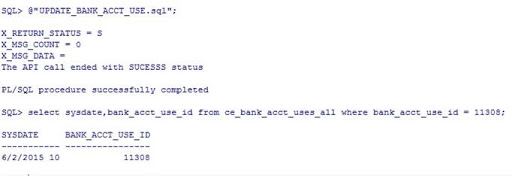# Script:

DECLARE

p_init_msg_list VARCHAR2(200);

p_acct_use_rec  apps.ce_bank_pub.bankacct_use_rec_type;

p_use_ovn       NUMBER;

p_ccid_ovn      NUMBER;

x_return_status VARCHAR2(200);

x_msg_count     NUMBER;

x_msg_data      VARCHAR2(200);

l_msg           VARCHAR2(200);

lv_asset_ccid   NUMBER;

lv_ar_use_flag  VARCHAR2(10);

lv_ap_use_flag  VARCHAR2(10);

BEGIN

-- Initialize apps session

fnd_global.apps_initialize(1234, 50833, 200);

mo_global.init('SQLAP');

fnd_client_info.set_org_context(101);

lv_ar_use_flag := 'Y';

lv_ap_use_flag := 'Y';

-- required for AP and AR use

-- SELECT asset_code_combination_id from CE_BANK_ACCOUNTS

-- WHERE bank_account_name = 'TEST'

lv_asset_ccid := 14944;

-- Assign API parameters

p_init_msg_list                          := fnd_api.g_true;

p_acct_use_rec.bank_acct_use_id          := 11308;

p_acct_use_rec.end_date                  := SYSDATE;

p_acct_use_rec.ap_use_enable_flag        := lv_ap_use_flag;

p_acct_use_rec.ar_use_enable_flag        := lv_ar_use_flag;

p_acct_use_rec.asset_code_combination_id := lv_asset_ccid;

-- NOT NULL COLUMNS

p_acct_use_rec.authorized_flag      := 'Y';

p_acct_use_rec.default_account_flag := 'N';

-- validated against ce_bank_acct_uses_all

p_use_ovn := 1;

-- Validated against ce_gl_accounts_ccid

p_ccid_ovn := 1;

-- Call the API CE_BANK_PUB.UPDATE_BANK_ACCT_USE

ce_bank_pub.update_bank_acct_use(p_init_msg_list => p_init_msg_list,

p_acct_use_rec  => p_acct_use_rec,

p_use_ovn       => p_use_ovn,

p_ccid_ovn      => p_ccid_ovn,

x_return_status => x_return_status,

x_msg_count     => x_msg_count,

x_msg_data      => x_msg_data);

DBMS_OUTPUT.put_line('X_RETURN_STATUS = ' || x_return_status);

DBMS_OUTPUT.put_line('X_MSG_COUNT = ' || x_msg_count);

DBMS_OUTPUT.put_line('X_MSG_DATA = ' || x_msg_data);

IF (x_return_status <> fnd_api.g_ret_sts_success) THEN

FOR i IN 1 .. x_msg_count LOOP

l_msg := fnd_msg_pub.get(p_msg_index => i,

p_encoded   => fnd_api.g_false);

DBMS_OUTPUT.put_line('The API call failed with error ' || l_msg);

END LOOP;

ELSE

DBMS_OUTPUT.put_line('The API call ended with SUCESSS status');

END IF;

END;

# Test Results:### 5 Responses to “API to update Internal Bank Account Uses in Oracle Apps R12 (CE_BANK_PUB.UPDATE_BANK_ACCT_USE)”

Unknown said...

I have a problem that the API shows the error : Cash GL Account is required. I would be grateful if you'll help.

Unknown said...

Regards
Sridevi Koduru (Senior Oracle Apps Trainer Oracleappstechnical.com)

Unknown said...

Thanks and Regards. Oracle Apps R12 Training Videos at affordable cost. please check oracleappstechnical.com

Anonymous said...

i am getting the following error message,

"The record has been updated by another user. (TABLE=CE_BANK_ACCT_USES_ALL)"

whereas in actual there is no session lock or any such thing on this table

Anonymous said...

Hello, I ave the same problem when calling api from DB or Oracle Forms ... Responses to “API to update Internal Bank Account Uses in Oracle Apps R12 (CE_BANK_PUB.UPDATE_BANK_ACCT_USE)” How did you solve? can you help me please?